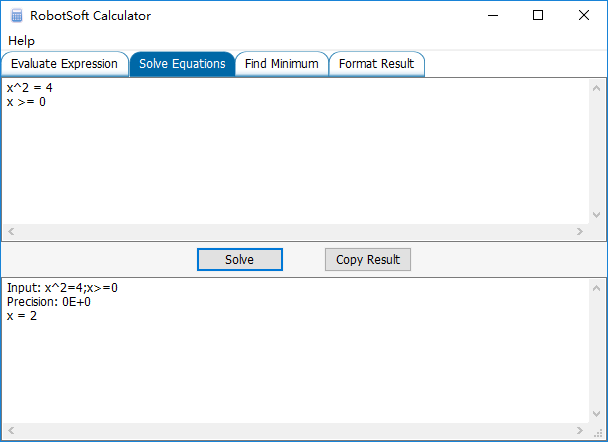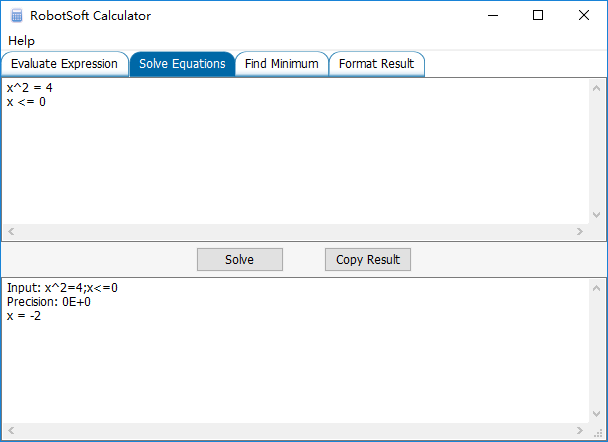RobotSoft Calculator now supports Greater Equal and Less Equal command

Uncategorized Comments Off on RobotSoft Calculator now supports Greater Equal and Less Equal command
Nov 192016

The Solve Equations feature of RobotSoft Calculator now supports Greater Equal and Less Equal command. Different conditions may get different results.

Assume x >= 0:Assume x <= 0:© 2021 RobotSoft Suffusion theme by Sayontan Sinha